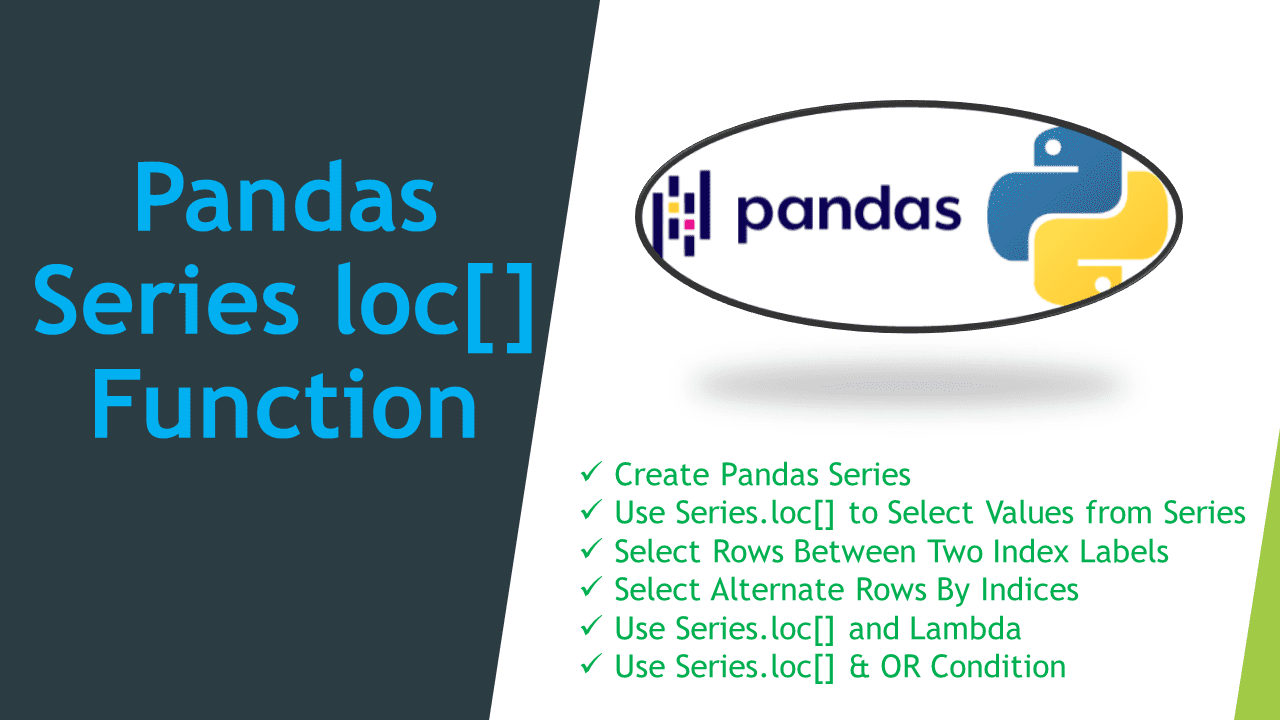# Pandas Series loc[] Function

• Post author:
• Post category:Pandas

Pandas `Series.loc[]` function is used to access a group of rows and columns by labels or a boolean array in the given Series object. We can select some values from the given Pandas Series object based on the labels using `loc[]` function. In this article, I will explain `Series.loc[]` to select Series object values by index labels.

## 1. Quick Examples of Series loc[] Function

If you are in hurry below are some quick examples of the Pandas Series `loc[]` function.

``````
# Below are quick examples.

# Examples 1: Use Series.loc[] function to selected labels
ser2 = ser.loc[['Pandas', 'Spark', 'Python']]

# Examples 2: Select Rows Between two Index Labels
# Includes both Spark and Python rows
ser2 = ser.loc['Spark':'Python']

# Examples 3: Select Alternate rows by indeces
ser2 = ser.loc['Spark':'Pandas':2]

# Examples 4: Use loc[] and lambda
ser2 = ser.loc[lambda x : x == 28000]

# Examples 5: Use loc[] property & OR condition
ser2 = ser.loc[lambda x : (x  28000)]
``````

## 2. Create Pandas Series

Pandas Series is a one-dimensional, Index-labeled data structure available only in the Pandas library. It can store all the datatypes such as strings, integers, float, and other python objects. We can access each element in the Series with the help of corresponding default indices.

Now, let’s create pandas series using list of values.

``````
import pandas as pd

# Create the Series
ser = pd.Series([20000,25000,23000,28000,55000,23000,28000])

# Create the Index
index = ['Java','Spark','PySpark','Pandas','python NumPy','Python',"Oracle"]

# Set the index
ser.index = index
print(ser)
``````

Yields below output.

``````
# Output:
Java            20000
Spark           25000
PySpark         23000
Pandas          28000
python NumPy    55000
Python          23000
Oracle          28000
dtype: int64
``````

## 3. Use Series.loc[] to Select Values from Series

You can use the Pandas `Series.loc[]` function to get or select the single value by Index or multiple values by index by specifying the index labels (in a list) from the Series object.

``````
# Use Series.loc[] function to selected labels
ser2 = ser.loc[['Pandas', 'Spark', 'Python']]
print(ser2)
``````

Yields below output.

``````
# Output:
Pandas    28000
Spark     25000
Python    23000
dtype: int64
``````

## 4. Select Rows Between Two Index Labels

loc[] also supports selecting rows or values between two index labels by range in pandas Series. For example, all items between starting and ending index. The below example selects rows between `Spark` and `Python`.

``````
# Select Rows Between two Index Labels
# Includes both Spark and Python rows
ser2 = ser.loc['Spark':'Python']
print(ser2)
``````

Yields below output.

``````
# Output:
Spark           25000
PySpark         23000
Pandas          28000
python NumPy    55000
Python          23000
dtype: int64
``````

## 5. Select Alternate Rows By Indices

Similarly, by using ranges you can also select every alternate row from Series. In order to do so, you need to provide the third value as a step.

``````
# Select Alternate rows by indeces
ser2 = ser.loc['Spark':'Pandas':2]
print(ser2)
``````

Yields below output.

``````
# Output:
Spark     25000
Pandas    28000
dtype: int64
``````

# 6. Use Series.loc[] and Lambda

You can also use Pandas `Series.loc[]` along with `lambda` function. The following example returns values from a series where values are equal to `28000`.

``````
# Use loc[] and lambda
ser2 = ser.loc[lambda x : x == 28000]
print(ser2)
``````

Yields below output.

``````
# Output:
Pandas    28000
Oracle    28000
dtype: int64
``````

## 7. Use Series.loc[] & OR Condition

You can also apply a `“OR”` condition with the `“loc[]”` property. The following example returns the values that are less than 25000 or values greater than 28000. For examples.

``````
# Use loc[] property & OR condition
ser2 = ser.loc[lambda x : (x  28000)]
print(ser2))
``````

Yields below output.

``````
# Output:
Java            20000
PySpark         23000
python NumPy    55000
Python          23000
dtype: int64
``````

## 8. Complete Examples of Pandas Series loc

``````
import pandas as pd

# Create the Series
ser = pd.Series([20000,25000,23000,28000,55000,23000,28000])

# Create the Index
index = ['Java','Spark','PySpark','Pandas','python NumPy','Python',"Oracle"]

# Set the index
ser.index = index
print(ser)

# Use Series.loc[] function to selected labels
ser2 = ser.loc[['Pandas', 'Spark', 'Python']]
print(ser2)

# Select Rows Between two Index Labels
# Includes both Spark and Python rows
ser2 = ser.loc['Spark':'Python']
print(ser2)

# Select Alternate rows by indeces
ser2 = ser.loc['Spark':'Pandas':2]
print(ser2)

# Use loc[] and lambda
ser2 = ser.loc[lambda x : x == 28000]
print(ser2)

# Use loc[] property & OR condition
ser2 = ser.loc[lambda x : (x  28000)]
print(ser2)
``````

## 9. Conclusion

In this article, you have learned about pandas Series loc property. `Series.loc[]` is label-based to select some values from the given Series object in pandas with examples.

Happy Learning !!

## References

### Naveen

I am a Data Engineer with 20+ years of experience in transforming data into actionable insights. Over the years, I have honed my expertise in designing, implementing, and maintaining data pipelines with frameworks like Apache Spark, PySpark, Pandas, R, Hive and Machine Learning. My journey in the field of data engineering has been a continuous learning, innovation, and a strong commitment to data integrity. I have started this SparkByExamples.com to share my experiences with the data as I come across. You can learn more about me at LinkedIn